### Path and Point Function

Work and Heat Transfer - is Path or Point Function

Work Transfer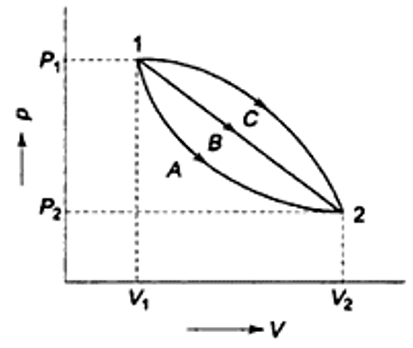Figure: p-V Diagram

With reference to figure it is possible to take a system from state 1 to state 2 along many quasi static paths such as A, B and C. Since the area under each curve represents the work for each process. The amount of work involved in each case is not a function of the end states of the process and it depends on the path the system follows going from state 1 to state 2. For this reason Work Transfer is called a Path Function and ᵭW is an inexact or imperfect differential.
It is given by-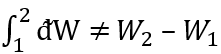Heat Transfer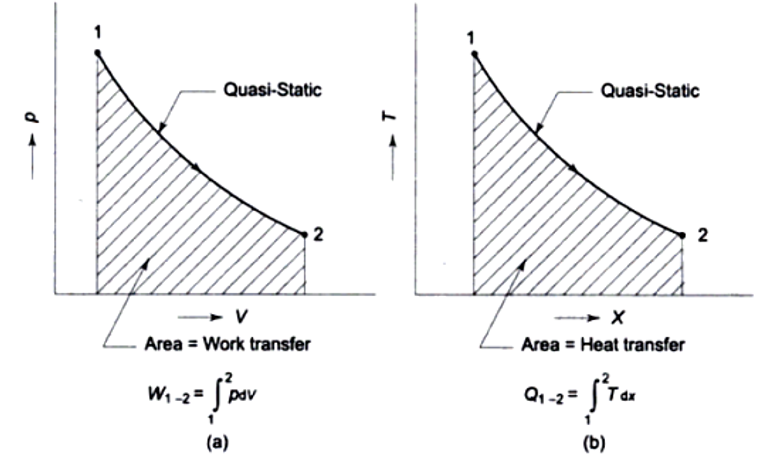Figure: Work Transfer on p-V Diagram and Heat Transfer on T-x Diagram

Heat transfer is the amount of heat transferred when a system changes from state 1 to state 2 depending on the intermediate states through which
the system passes. Therefore ‘ᵭQ’ is an inexact differential and we can write as-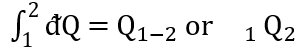Whenever there is a difference in temperature, there will be heat flow. The difference is the cause and heat transfer is the effect. The heat transfer is
the integral of the product of intensive property ‘T’ and differential change of an extensive property ‘x’ as shown in figure.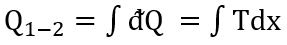The heat transfer involved is represented by the area under the path 1-2 in T-x plot as in figure. Heat transfer is therefore a Path Function.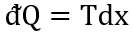To make ‘ᵭQ’ an exact differential it must be multiplied by an integrating factor ‘1/T’.